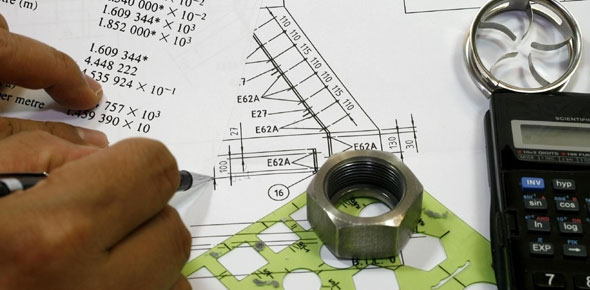# All India Barc Online Test In Mechanical Engg.

100 Questions | Attempts: 304Settings.

• 1.
Which one of the following materials is used as the bonding material for grinding wheels
• A.

Silicon carbide

• B.

Sodium silicate

• C.

Boron carbide

• D.

Aluminium oxide

• 2.
In reaming process
• A.

The metal removal rate is high

• B.

High surface finish is obtained

• C.

High form of accuracy is obtained

• D.

High degree of tolerance achieved

• 3.
In the griding wheel have specification A60G7B23, B stands for the
• A.

Resinoid bond

• B.

Rubber bond

• C.

Shellac bond

• D.

Silicate bond

• 4.
• A.

Types of wheel bond fracture

• B.

Types of wheel grain fracture

• C.

Sticking of small particle on grinding wheel

• D.

None of the above

• 5.
Helix angle of a fast helix drill is normally
• A.

35

• B.

60

• C.

90

• D.

5

• 6.
A milling cutter having 8 teeth is rotating at 150 rpm. if the feed per tooth is 0.1 mm, the value of thew table speed in mm/min is
• A.

120

• B.

187

• C.

125

• D.

70

• 7.
Gas having index of expansion is 2 flows through convergent nozzle the Critical pressure ratio is
• A.

0.66 bar

• B.

0.44 bar

• C.

0.1975 bar

• D.

0.81 bar

• 8.
Among the conventional, machining process maximium specifiec energy is consumed in
• A.

Grinding

• B.

Drilling

• C.

Planing

• D.

Turnig

• 9.
The enthalpy of steam at the inlet and outlet of a steam turbine is 2850 KJ/Kg and 1950 KJ/Kg respectively. The specifiec steam consumption in Kg/KW hour is
• A.

4

• B.

3

• C.

5

• D.

2

• 10.
The Rateau Turbine belongs to the category of
• A.

Pressure compounded turbine

• B.

Reaction turbine

• C.

Velocity compounded turbine

• D.

• 11.
For a single stage impulse turbine with rotor diameter of 2 m and a speed of 3000 rpm when the nozzle angle is 20 Degree, The Optimium velocity of steam in m/s
• A.

334

• B.

356

• C.

668

• D.

711

• 12.
The bottles from thermo plastic materials are made by
• A.

Compression moulding

• B.

Extrusion with mandrel

• C.

Injection moulding

• D.

blow moulding

• 13.
The process of producing components in moulds without the application of pressure, is known as
• A.

Moulding

• B.

Laminating

• C.

Casting

• D.

Calendering

• 14.
A Gas Turbine Cycle with heat exchange and reheating improves
• A.

Only the thermal efficiency

• B.

Only the Specific power output

• C.

Both thermal effiecincy and specific power output

• D.

Any one of the above

• 15.
In powder metallurgy, the operation carried out to improve the bearing property of a bush is called
• A.

Infilteration

• B.

Impregnation

• C.

Plating

• D.

Sintering

• 16.
Constant Pressure lines in the superheated region of the Mollier Diagram will have
• A.

A positive slope

• B.

A negative slope

• C.

Zero slope

• D.

Asymptote

• 17.
Cold shortness is defined as the
• A.

Fracture in room temperature below their recrystallization temperature

• B.

Brittelness in room temperature below their recrystallization temperature

• C.

Loss of ductility above recrystallization temperature

• D.

Decrease of brittleness in room temperature

• 18.
The compositions of some of the alloy steels are as under. Which of the following is Correct Designation
• A.

18 W 4 Cr 1 V

• B.

12 Mo 1 W 4 Cr 1 V

• C.

6 Mo 6 W 4 Cr 1 V

• D.

18 W 8 Cr 1 V

• 19.
A Heat reservior at 1000 K is brought into contact with another Reservior at 400 K for 2 Second. During this Period 9000 KJ of heat is lost by the heat reservior. The Change in Entropy (KJ/K) due to temperature difference
• A.

20

• B.

40

• C.

30

• D.

60

• 20.
A Carnot cycle is having of 0.75. if the temperature of the high temperature reservior is 1000 K. What is the temperature of low temperature reservior
• A.

250 K

• B.

300 K

• C.

350 K

• D.

400 K

• 21.
A Grinding wheel of 150mm diameter is rotating at 3000 rpm. The grinding speed is
• A.

7.5 π m/s

• B.

15π m/s

• C.

45π m/s

• D.

450π m/s

• 22.
In Certain type of Heat Exchanger , Both the fluids have identical mass flow rate Specific heat product. The hot fluid enters at 349 K and leaves at 322 K and the cold fluid entering at 301 K leave at 328 K. The effectiveness of HE IS
• A.

0.7

• B.

0.6

• C.

0.2

• D.

0.3

• 23.
A Lead Screw with half nuts in a lathe, Free to Rotate in both Direction has
• A.

• B.

• C.

• D.

• 24.
A Car moving with uniform acceleration covers 700 m in the next 5 second. The acceleration of the car in m/s^2.
• A.

20

• B.

10

• C.

30

• D.

40

• 25.
In Highly rarefied gases, the concept loses validity is associated with
• A.

Continum Theory

• B.

Thermodynamic Equilibrium

• C.

Stability

• D.

Macroscopic Approach

## Related TopicsBack to top
×

Wait!
Here's an interesting quiz for you.